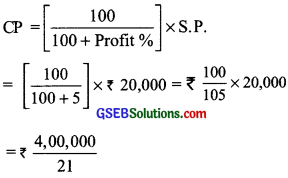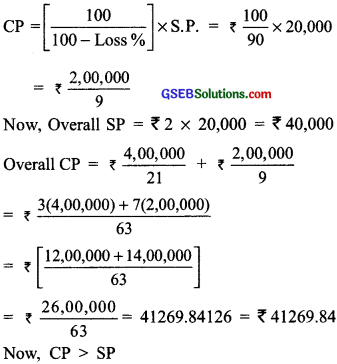# GSEB Solutions Class 8 Maths Chapter 8 Comparing Quantities Ex 8.2

Gujarat Board GSEB Textbook Solutions Class 8 Maths Chapter 8 Comparing Quantities Ex 8.2 Textbook Questions and Answers.

## Gujarat Board Textbook Solutions Class 8 Maths Chapter 8 Comparing Quantities Ex 8.2

Question 1.
A man got a 10% increase in his salary. If his new salary is ₹ 1,54,000, find his original salary?
Solution:
Let the original salary be ₹ x
∴ Increase in salary = 10% of ₹ x
= ₹ $$\frac{10}{100}$$x = ₹ $$\frac{x}{10}$$
New salary = ₹ x + ₹ $$\frac{x}{10}$$ = ₹ $$\frac{11}{10}$$ x
∴ ₹ $$\frac{11}{10}$$x = ₹ 154000
or x = ₹ $$\frac{154000×10}{11}$$ = ₹ 1,40,000
Thus, the original salary = ₹ 1,40,000Question 2.
On Sunday 845 people went to the Zoo. On Monday only 169 people went. What is the per cent decrease in the people visiting the Zoo on Monday?
Solution:
Number of visitors on Sunday = 845
Number of visitors on Monday = 169
Decrease in the number of visitors = 845 – 169 = 676
∴ Per cent decrease = $$\frac{676}{845}$$ × 100%
= (4 × 20)% = 80%

Question 3.
A shopkeeper buys 80 articles for ₹ 2,400 and sells them for a profit of 16%. Find the selling price of one article?
Solution:
Cost price = ₹ 2400
Profit = 16% of ₹ 2400 = ₹ $$\frac{16}{100}$$ × 2400
= ₹ 16 × 24 = ₹ 384
∴ Selling price = ₹ 2400 + ₹ 384 = ₹ 2784
Now, selling price per article = ₹ 2784 + 80
[∵ Number of articles = 80] = ₹ 34.80Question 4.
The cost of an article was ₹ 15,500. ₹ 450 were spent on its repairs. If it is sold for a profit of 15%, find the selling price of the article?
Solution:
Total cost = ₹ 15500 + ₹ 450 (Overhead expenses)
= ₹ 15950
Profit = 15% of ₹ 15950
= ₹ $$\frac{15}{100}$$ × 15950
= ₹ $$\frac{15}{100}$$ × 15950 = ₹ $$\frac{3×1595}{2}$$
= ₹ $$\frac{4785}{2}$$ = ₹ 2392.50
∴ Selling price = ₹ 15950 + ₹ 2392.50 = ₹ 18342.50

Question 5.
A VCR and TV were bought for ₹ 8,000 each. The shopkeeper made a loss of 4% on the VCR and a profit of 8% on the TV. Find the gain or loss per cent on the whole transaction?
Solution:
For VCR
CP = ₹ 8000
Loss = 4% of ₹ 8000 = ₹ $$\frac{4}{100}$$ × 8000 = ₹ 320
∴ SP = ₹ 8000 – ₹ 320 = ₹ 7680
Now, total CP = ₹ 8000 + ₹ 8000 = 16000 For TV
CP = ₹ 8000
Profit = 8% of ₹ 8000 = ₹ $$\frac{8}{100}$$ × 8000 = ₹ 640
SP = ₹ 8000 + ₹ 640 = ₹ 8640
Total SP = ₹ 7680 + ₹ 8640 = ₹ 16320
∵ SP > CP
∴ Overall profit = ₹ 16320 – ₹ 16000 = ₹ 320
Overall profit per cent = $$\frac{320}{16000}$$ × 100% = 2%Question 6.
During a sale, a shop offered a discount of 10% on the marked prices of all the items. What would a customer have to pay for a pair of jeans marked at ₹ 1450 and two shirts marked at ₹ 850 each?
Solution:
For a pair of Jeans
Marked price = ₹ 1450
Discount = 10% of ₹ 1450 = ₹ $$\frac{10}{100}$$ × 1450 = ₹ 145
∴ Sale price = ₹ 1450 – ₹ 145 = ₹ 1305 For two Shirts
Marked price (₹ 850) × 2 = ₹ 1700
Discount = 10% of ₹ 1700
= ₹ $$\frac{10}{100}$$ × 1700 = ₹ 170
∴ Sale price = ₹ 1700 – 170 = 1530
Total sale price = ₹ 1305 + ₹ 1530 = ₹ 2835
Thus, the customer would have to pay ₹ 2835.

Question 7.
A milkman sold two of his buffaloes for ₹ 20,000 each. On one he made a gain of 5% and on the other a loss of 10%. Find his overall gain or loss. (Hint: Find CP of each.)
Solution:
For 1st buffalo:
SP = ₹ 20,000 Profit = 5%For 2nd buffalo:
SP = ₹ 20,000 Loss = 10%∴ Overall loss = ₹ (41269.84 – 4000)
= ₹ 1269.84Question 8.
The price of a TV is ₹ 13,000. The sales tax charged on it is at the rate of 12%. Find the amount that Vinod will have to pay if he buys it?
Solution:
Cost (sale price) of the TV = ₹ 13,000
Rate of sales tax = 12%
∴ Sales tax= 12% of ₹ 13000 = ₹ $$\frac{12}{100}$$ × 13000
= ₹ (12 × 130) = ₹ 1560
∴ Bill amount = ₹ 13000 + ₹ 1560 = ₹ 14,560
Thus Vinod will have to pay ₹ 14,560 for the TV.

Question 9.
Arun bought a pair of skates at a sale where the discount given was 20%. If the amount he pays is ₹ 1,600, find the marked price?
Solution:
Let the marked price = ₹ 100
∴ Discount = 20% of ₹ 100
= ₹ $$\frac{20}{100}$$ × 100 = ₹ 20
Sale price = ₹ (100 – 20) = ₹ 80
If sale price is ₹ 80, then marked price = ₹ 100
If sale price is ₹ 1600, then marked price
= ₹ $$\frac{100}{80}$$ × 1600 = ₹ 2000Question 10.
I purchased a hair-dryer for ₹ 5,400 including 8% VAT. Find the price before VAT was added?
Solution:
Let the original pricebe ₹ 100
∴ Original price + VAT
= ₹ 100 + ₹ (8% of 100)
= ₹ 100 + ₹ ($$\frac{8}{100}$$ × 100) = ₹ 108
∴ Bill amount = ₹ 108
If bill amount is ₹ 108, then original price = ₹ 100
If bill amount is ₹ 5,400, then original price
= ₹ $$\frac{100}{108}$$ × 5400 = ₹ (100 × 50) = ₹ 5000